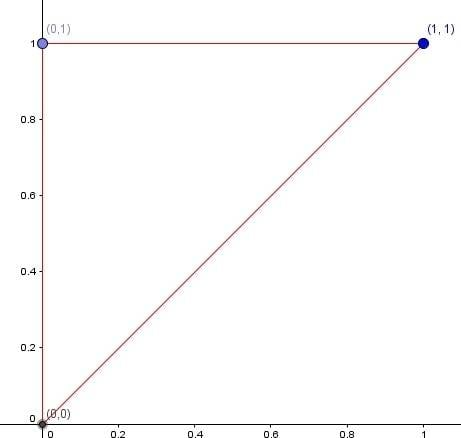# Integration of a triangle

says

## Homework Statement

Evaluate ∫∫D sin(x) / x dA, where A is the triangle with vertices (0, 0),(0, 1),(1, 1).

## The Attempt at a Solution

I've set up the integral, with x≤y≤x^2, 0≤x≤1 for the upper and lower bands of each integral. dxdy
I'm not sure how to go about it though.
I can re-write the integral to be sin(x)*x-1 and do integration by parts but I get stuck in a loop. Is there some method of integration I should be using instead?

Homework Helper
Gold Member

## Homework Statement

Evaluate ∫∫D sin(x) / x dA, where A is the triangle with vertices (0, 0),(0, 1),(1, 1).

## The Attempt at a Solution

I've set up the integral, with x≤y≤x^2, 0≤x≤1 for the upper and lower bands of each integral. dxdy
I'm not sure how to go about it though.
What does ##y=x^2## have to do with this problem? Try setting it up as a dydx integral with corrected limits.

•says
Brian T
As said above, you need to use the correct limits. It is not good enough to choose limits which agree at the boundaries (which yours doesn't even do), you need to have the correct domain throughout (e.g. for a triangle use linear functions).

•says
says
ok, so for dydx
x≤y≤1
0≤x≤y

says
sin(x)/x cannot be integrated.
If I set up the integral with the correct regions, which I think they are in the post above, I still won't be able to integrate it, no?

Homework Helper
ok, so for dydx
x≤y≤1
0≤x≤y
The integration limits for the outer integral (over x), can't contain a y.
sin(x)/x cannot be integrated.
If I set up the integral with the correct regions, which I think they are in the post above, I still won't be able to integrate it, no?
Even with correct regions, I'm not sure that this integral can be easily computed.
Are you sure about the three points? Maybe it should be (1,0) and not (0,1).

•says
says
But the rightmost value of x=y and the leftmost value of x=0
The highest value of y=1 and the lowest value of y=x
x≤y≤1
0≤x≤y

Is this not saying the same thing as:
0≤y≤1
0≤x≤1

?
I wrote in x and y into my first region because I thought it had to be more general. i.e. if we changed the points to be say, (0,0), (0,5) (5,5) we could say the region is:

x≤y≤5
0≤x≤y

or do we just put the actual values in the domain? So it would be:

0≤y≤5
0≤x≤5

says
I think I got the limit of integration for y, just by rewriting it. I can now compute the first integral.
0 ≤ y ≤ x

says
I think I got the limit of integration just by rewriting it. I can now compute the first integral.
0 ≤ y ≤ x
0 ≤ x ≤ 1

∫sin(x)/x dy
=sin(x)y/x
| evaluated at 0 ≤ y ≤ x
=sin(x)
∫sin(x) dx
= -cos(x)
| evaluated at 0 ≤ x ≤ 1
=1-cos(1)

I'm still having trouble setting up limits of integration. We had 3 points of the triangle, and (1,1) was the highest point for y, so how come I can't say 0 ≤ y ≤ x ? I guess I could say that, but it wouldn't get me very far computing the integral. Is that really all there is to it? Looking at the problem more and trying to find equivalent statements?

Homework Helper
Don't guess the integration limits. Draw your triangle, and try to deduce them.
This is your triangle (if the points are indeed as you posted in post #1):Since you first integrate over y, fix x, and determine what values of y are relevant. That will give you the limits for y, and these limits can depend on x.
Then determine the limits for x. These can't depend on y.

•says
Homework Helper
I think I got the limit of integration just by rewriting it. I can now compute the first integral.
0 ≤ y ≤ x
0 ≤ x ≤ 1
These limits correspond to the triangle defined by the points (0,0), (1,0), (1,1). Not the points you stated in post #1.

•blue_leaf77 and says
says
Damn! The points are as stated in post #1

says
Argh! Just checked my email -- teacher said to correct the point to (1,0). ARGH!

Thanks again for your help, Samy_A!

•